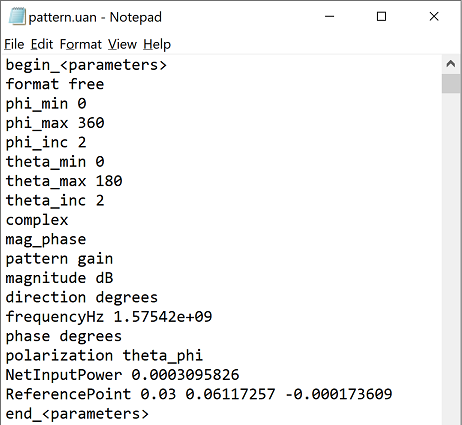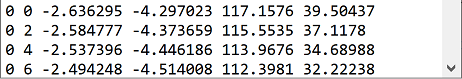A user-defined antenna (UAN) file contains antenna pattern information in text format that users can create and read for use in post-processing applications. The *.uan file format is unique to Remcom and ideal for sharing data across its suite of design and electromagnetic simulation products, including XFdtd®, Wireless Insite®, XGtd®, WaveFarer®.

## UAN File StructureAlthough the *.uan file supports multiple formats, XF writes the complex output in one format only. The *.uan file begins with text concerning parameters. The theta-phi coordinate system provides definitions relative to the x-, y-, and z-axes.

The format free text line is of no significance to the file's content, but explanations for the remaining lines are as follows:

• phi_min: minimum phi observation angle in degrees.
• phi_max: maximum phi observation angle in degrees.
• phi_inc: phi observation angle increment in degrees.
• theta_min: minimum theta observation angle in degrees.
• theta_max: maximum theta observation angle in degrees.
• theta_inc: theta observation angle increment in degrees.
• complex: data consists of complex numbers.
• mag_phase: data is magnitude and phase.
• pattern gain: quantity being stored is gain.
• magnitude dB: data magnitude component is dB.
• direction degrees: observation angles are specified in degrees.
• frequencyHz: frequency of data in Hz.
• phase degrees: data phase component is degrees.
• polarization theta_phi: polarization for antenna radiation measurements.
• NetInputPower: simulation's net input power in watts.
• ReferencePoint: far zone pattern's phase reference point as x, y, and z coordinates in meters.The parameters portion is followed by a data section that provides angle-based pattern data of one row per angle.

The following six variables correspond to the six columns in the data portion of the *.uan file:

\begin{eqnarray} \theta\,\,\,\,\phi\,\,\,\,G_{\theta}(\theta,\phi)\,\,\,\,G_{\phi}(\theta,\phi)\,\,\,\,\angle{E_{\theta}(\theta,\phi)}\,\,\,\,\angle{E_{\phi}(\theta,\phi)} \end{eqnarray}

where $\theta$ is the theta observation angle in degrees, $\phi$ is the phi observation angle in degrees, $G_{\theta}(\theta,\phi)$ is the theta polarized gain in dB, $G_{\phi}(\theta,\phi)$ is the phi polarized gain in dB, $\angle{E_{\theta}(\theta,\phi)}$ is the theta polarized E-field phase in degrees, and $\angle{E_{\phi}(\theta,\phi)}$ is the phi polarized E-field phase in degrees.

## Convert Gain to E-Field

E-field magnitude is not provided in a *.uan file, but it is determined by first converting gain in dB to linear units

\begin{equation} G_{linear}=10^\frac{G_{dB}}{10} \end{equation}

and then converting gain to E-field magnitude

\begin{equation} |E|=\sqrt{\frac{G_{linear}\eta_0 P_{in}}{2\pi}} \end{equation}

where $\eta_0$ is the impedance of free space and $P_{in}$ is the NetInputPower available in the parameters section of the *.uan file.